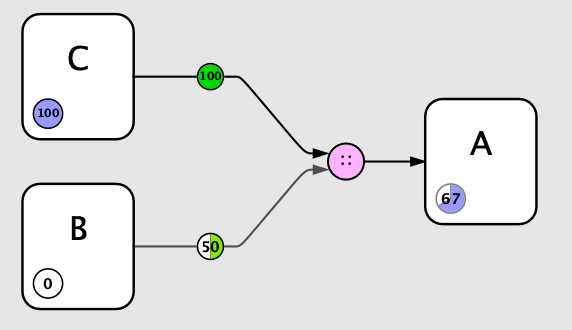# How to calculate "Proportion"?

When it comes to the “Proportion” junctor, the “Flying Logic User Guide” says: “Treats each input as a “vote” of a strength proportional to the confidence value and the edge weight. Edge weights of zero count as abstentions and do not affect the output, which is different from a simple average where each zero input tends to reduce the output. Output value is fuzzy boolean unless at least one input is floating-point, in which case the output is floating-point.”. However, it’s still unclear to me how is the result calculated. Can you give us the formula?
For example, let’s assume you have an input entity of “True” injecting (+) at 100% weight. And another input entity of “False” injecting (+) at 50% weight. Both of them enter the junctor ∷ (proportion). What is the value of the confidence on the resulting entity, and how exactly is calculated? It seems the result is something like 66% True, but I’m not sure and I don’t know how exactly is calculated. Also, the result is only a “Confidence circle”. It has no clear value displayed. Can you clarify this for us? Thank you.

Here is pseudocode for how the Proportion operation works:

For a given set of `input`s (incoming edges), each of which has a confidence `value` in [0, 1] and a `weight` in [-1, 1]:

``````let totalAbsWeight = sum of the absolute value of each input weight
if totalAbsWeight ≈ 0 {
return 0
} else {
let totalValue = sum of all input values
}
``````

So for your example: first input (value: 1, weight: 1), second input (value: 0, weight 0.5).
totalAbsWeight = 1 + 0.5 == 1.5
totalValue = 1 + 0 == 1
result = totalValue / totalAbsWeight == 1 / 1.5 == 0.66~

Flying Logic also has a Preference to let you display numerical percentages ranged from [0…100]:

With that turned on you can see the result of the above example:Put into plain English: An analyst is polling B and C on proposition A. C believes it to be true, so that confidence spinner is set to 100%. B believes it to be false, so that spinner is set to 0%. The analyst then sets the edge weights to reflect an understanding of how much weight B and C’s vote should each be given, based on things like credentials or past performance. The aggregate opinion is a 67% probability of A being true.

Another way of looking at it is that confidence values are the presence or absence of factors that impact an outcome, and weights are how strongly they weigh for or against that outcome.

Flying Logic has a built-in example of the Proportion operation being used:

The Entity Operator in this document is set to Proportion.

You can find the document in File > Open Examples… > Belief Network > Hydrogen Production.

Thank you, Wolf.
I really appreciate you taking the time to answer this question at length. Very helpful. I understood the weight and confidence work in the proportion operation. I didn’t quite understand the formula. I’m in the process of learning all the operations with accuracy.
Virgil

1 Like